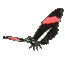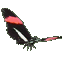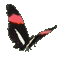Graphing Sine Waves - Trigonometry Help - Game Tips:

- Sine waves are used to model many periodic and vibrational patterns in nature.
- The equation y = A sin (kT)   represents a set of ordered pairs of the form (T,y).

- When graphed on a horizontal T-axis and vertical y-axis, the curve represented by y = A sin (kT)
is a Sine wave having vertical amplitude = |A| and horizontal wavelength = |2(Pi)/(k)|.

- In a vibrating string example, |A| is the maximum deflection of the string from rest position.
Wavelength refers to how long it takes for a pattern to repeat itself. Eg. a crest + a trough .
As 'k' gets larger in size, the wavelength gets shorter. Also Pi = 3.14159 approximately.

- Your Game Score is reduced by the number of butterfly hits.

- To slow the game speed repeat tap/click on the word Slider.
- To increase the game speed repeat tap/click on the word Math.
- Speed can also be adjusted with a keyboard's - and + keys.

- Refresh/Reload the web page to restart the game.